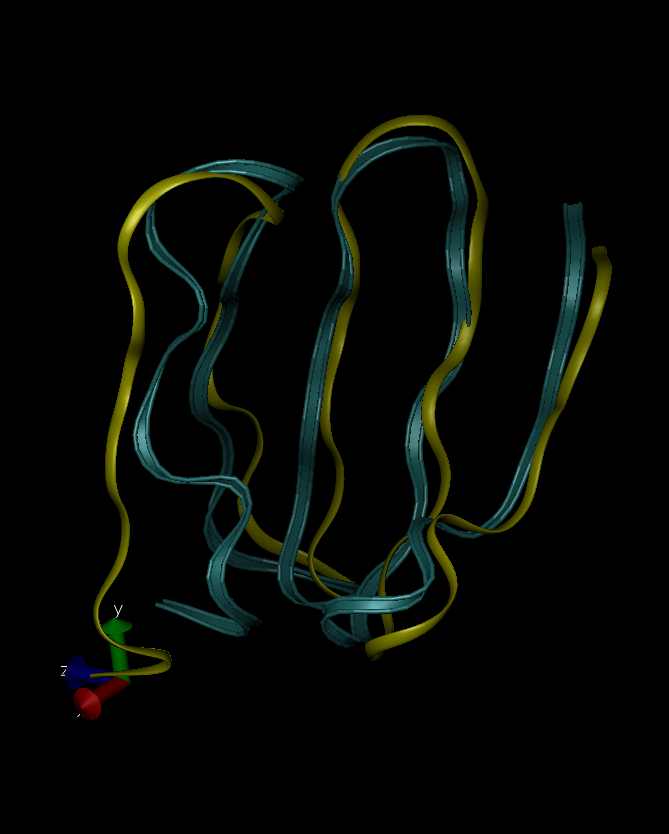lammps错误诊断8-Out of range atoms - cannot compute PPPM

2018/12/22 15:49

---------------- Step        0 ----- CPU =      0.0000 (sec) ----------------
TotEng   =           -nan KinEng   =         0.0000 Temp     =         0.0000
PotEng   =           -nan E_bond   =      5536.5700 E_angle  =           -nan
E_dihed  =       405.0252 E_impro  =       799.1751 E_vdwl   =           -nan
E_coul   =           -nan E_long   =     -2301.2059 Press    =           -nan
ERROR on proc 8: Out of range atoms - cannot compute PPPM (../pppm.cpp:1937)
ERROR on proc 15: Out of range atoms - cannot compute PPPM (../pppm.cpp:1937)

There is no secondary-structure information recorded.
Bond length is unusually long: 4.79754 223-222
Bond length is unusually long: 2.70009 328-327
Bond length to Hydrogen is unusually long: 2.13469 456-457
Bond length to Hydrogen is unusually long: 2.13561 456-458

Since there is no secondary-structure information, ChemBio3D Ultra is going to formcompute it.
The number of atoms in this molecule exceeds the atom synchronization limit. The ChemDraw panel cannot load the molecular structure.
MM2 Calculation completed successfully
------------MM2 Minimization------------
Warning: The number of ligands attached does not match the geometry of N(1)
Warning: The number of ligands attached does not match the geometry of O(139)
Warning: The number of ligands attached does not match the geometry of O(453)
Warning: The number of ligands attached does not match the geometry of C(457)
Warning: The number of ligands attached does not match the geometry of H(458)
Warning: The number of ligands attached does not match the geometry of H(459)
Warning: The number of ligands attached does not match the geometry of C(559)
Separating coincident atoms: C(63)-H(64)
Separating coincident atoms: C(238)-H(239)
Pi System:  538 536 534 533 541 543
Warning: Some parameters are guessed (Quality = 1).
Iteration    1  Steric Energy 274941803.943  RMS Gradient 1341504.134  RMS Move   0.0000
Iteration    2  Steric Energy 104634145.547  RMS Gradient 628201.522  RMS Move   0.0892
Iteration    3  Steric Energy 98644711.696  RMS Gradient 619854.158  RMS Move   0.0223
Iteration    4  Steric Energy 36519658.997  RMS Gradient 278060.506  RMS Move   0.0717
Iteration    5  Steric Energy 33726135.351  RMS Gradient 272796.212  RMS Move   0.0223
Iteration    6  Steric Energy 14471590.989  RMS Gradient 125631.455  RMS Move   0.0497
Iteration    7  Steric Energy 13180439.253  RMS Gradient 125367.907  RMS Move   0.0223
------------------------------------------------------------------
Iteration 6205  Steric Energy -157.182  RMS Gradient    0.015  RMS Move   0.0000
Iteration 6206  Steric Energy -157.182  RMS Gradient    0.011  RMS Move   0.0000
Iteration 6207  Steric Energy -157.182  RMS Gradient    0.009  RMS Move   0.0000
Iteration 6207: Minimization terminated normally because the gradient norm is less than the minimum gradient norm
Stretch:               25.2323
Bend:                 288.0828
Stretch-Bend:          -1.1579
Torsion:              141.6311
Non-1,4 VDW:         -380.2947
1,4 VDW:              148.6268
Dipole/Dipole:       -379.3025
Total Energy:          -157.1821 kcal/mol
Calculation ended---------------- Step        0 ----- CPU =      0.0000 (sec) ----------------
TotEng   =      6842.2689 KinEng   =         0.0000 Temp     =         0.0000
PotEng   =      6842.2689 E_bond   =      5217.8375 E_angle  =       889.2725
E_dihed  =       220.4900 E_impro  =         1.9840 E_vdwl   =       373.2779
E_coul   =      2417.1047 E_long   =     -2277.6976 Press    =    -10629.6498
---------------- Step       10 ----- CPU =      0.0337 (sec) ----------------
TotEng   =      2472.8837 KinEng   =         0.0000 Temp     =         0.0000
PotEng   =      2472.8837 E_bond   =      1476.8188 E_angle  =       611.9309
E_dihed  =       226.6634 E_impro  =         2.8918 E_vdwl   =        31.9095
E_coul   =      2405.3611 E_long   =     -2282.6917 Press    =     -3317.6592
---------------- Step       20 ----- CPU =      0.0562 (sec) ----------------
TotEng   =       998.0748 KinEng   =         0.0000 Temp     =         0.0000
PotEng   =       998.0748 E_bond   =       200.8747 E_angle  =       453.3712
E_dihed  =       234.2443 E_impro  =         6.1779 E_vdwl   =        -6.6921
E_coul   =      2397.4972 E_long   =     -2287.3984 Press    =      1691.6034

0
0 收藏

作者的其它热门文章0 评论
0 收藏
0